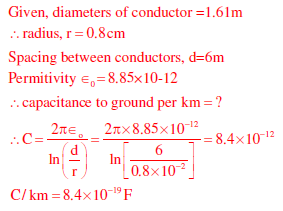# Power Systems - Online Test

Q1. The sequence components of the fault current are as follows: Ipositive = j1.5 pu, Inegative = –j0.5 pu, Izero = –j1 pu. The type of fault in the system is
Explaination / Solution:

I1 = I2 + I0

So LLG fault

Q2. A cylindrical rotor generator delivers 0.5 pu power in the steady-state to an infinite bus through a transmission line of reactance 0.5 pu. The generator no-load voltage is 1.5 pu and the infinite bus voltage is 1 pu. The inertia constant of the generator is 5 MW-s/MVA and the generator reactance is 1 pu. The critical clearing angle, in degrees, for a three-phase dead short circuit fault at the generator terminal is
Explaination / Solution:Q3. A single-phase load is supplied by a single-phase voltage source. If the current flowing from the load to the source is 10<− 150°A and if the voltage at the load terminals is 100<60°V , then the
Explaination / Solution:
No Explaination.

Q4. A source vs(t) = V cos 100πt has an internal impedance of (4 + j3)Ω. If a purely resistive load connected to this source has to extract the maximum power out of the source, its value in Ω should be
Explaination / Solution:
No Explaination.

Q5. When the Newton-Raphson method is applied to solve the equation f(x) = x3 + 2x – 1 = 0, the solution at the end of the first iteration with the initial guess value as x= 1.2 is
Explaination / Solution:
No Explaination.

Q6. For a power system network with n nodes, Z33 of its bus impedance matrix is j0.5 per unit. The voltage at node 3 is 1.3 ∠–10° per unit. If a capacitor having reactance of –j3.5 per unit is now added to the network between node 3 and the reference node, the current drawn by the capacitor per unit is
Explaination / Solution:
No Explaination.

Q7. In the following network, the voltage magnitudes at all buses are equal to 1 p.u., the voltage phase angles are very small, and the line resistances are negligible. All the line reactances are equal to j1 Ω.The voltage phase angles in rad at buses 2 and 3 are
Explaination / Solution:
No Explaination.

Q8. In the following network, the voltage magnitudes at all buses are equal to 1 p.u., the voltage phase angles are very small, and the line resistances are negligible. All the line reactances are equal to j1 Ω.If the base impedance and the line-to line base voltage are 100 ohms and 100kV respectively, then the real power in MW delivered by the generator connected at the slack bus is
Explaination / Solution:
No Explaination.

Q9. Shunt reactors are sometimes used in high voltage transmission system to
Explaination / Solution:
No Explaination.

Q10. The horizontally placed conductors of a single phase line operating at 50 Hz are having outside diameter of 1.6 cm, and the spacing between centers of the conductors is 6 m. The permittivity of free space is 8.854 × 10-12 F/m. The capacitance to ground per kilometer of each line is
Explaination / Solution: